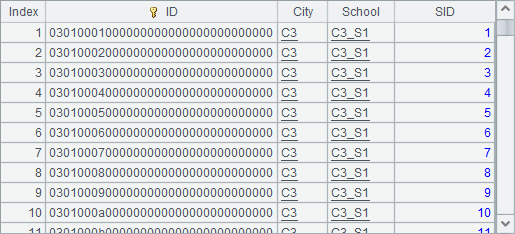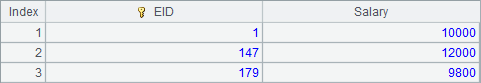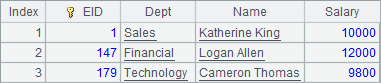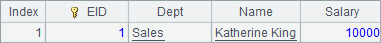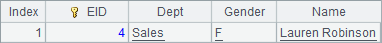# 柱图元

## 柱图元及其数据属性

 A 1 =canvas() 2 =demo.query("select * from GYMSCORE") 3 =A1.plot("BackGround") 4 =A1.plot("EnumAxis","name":"x") 5 =A1.plot("NumericAxis","name":"y","location":2) 6 =A1.plot("Column","axis1":"x","data1":A2.(EVENT+","+NAME),"axis2":"y","data2":A2.(SCORE)) 7 =A1.draw@p(450,200)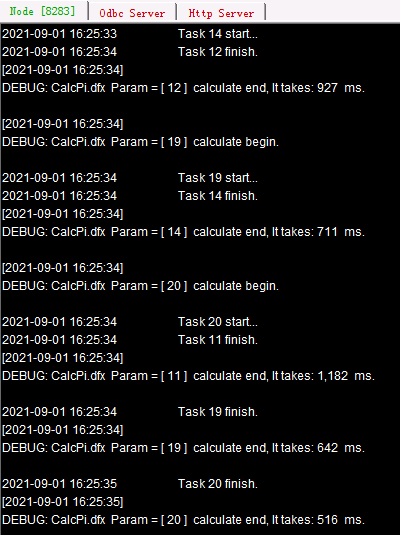A3绘制白色背景；A4中设定横轴x为枚举轴，自动分析数据；A5中设置纵轴y为数值轴，在这个画法中并未绘制图例。A6中绘制柱图，后面将详细分析柱图元的绘图属性。

A7中绘图结果如下：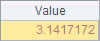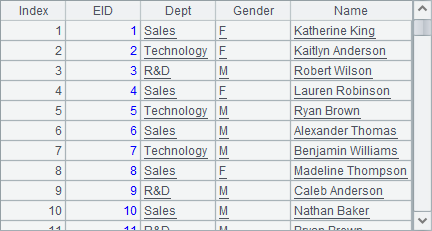## 柱图元的宽度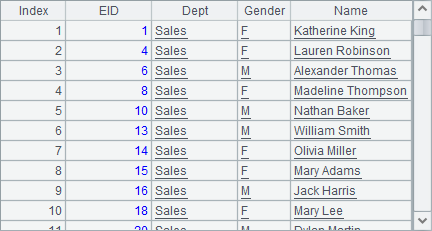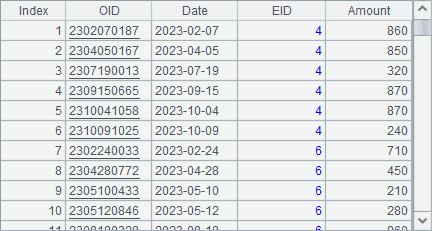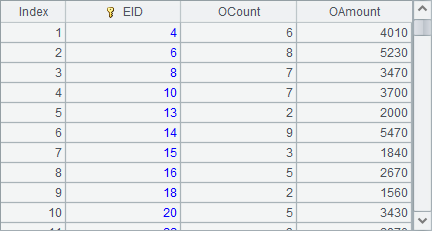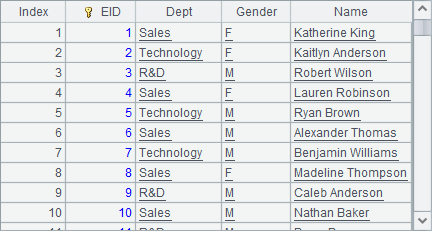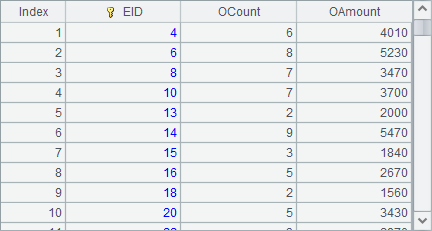## 柱图元的外观属性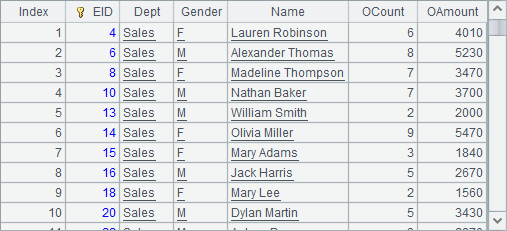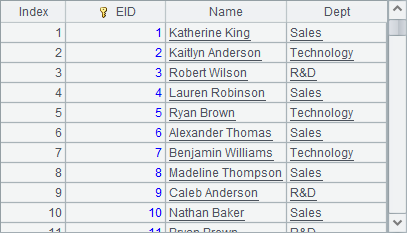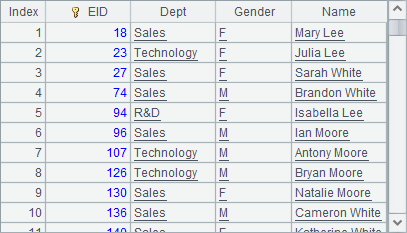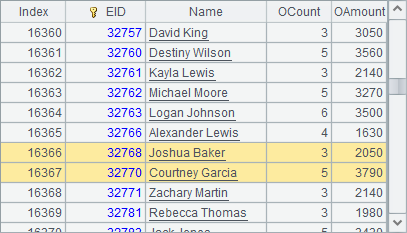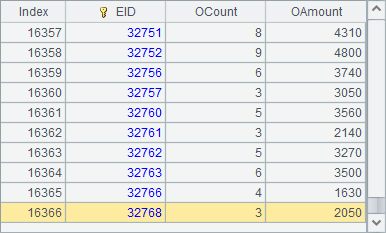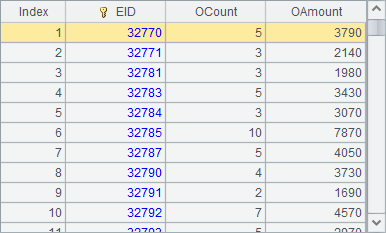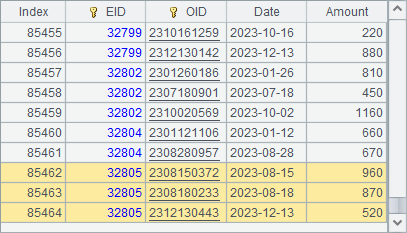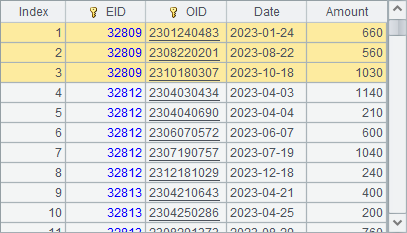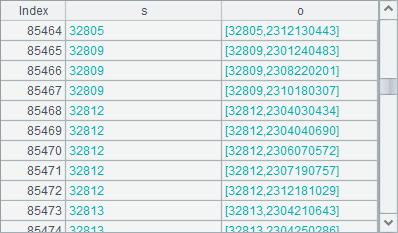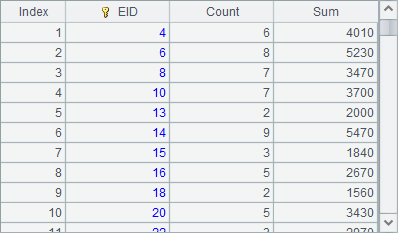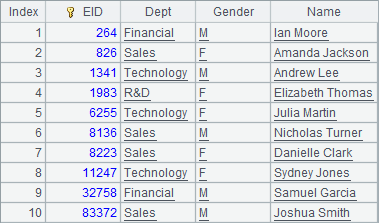## 堆积图

 A 1 =canvas() 2 =demo.query("select * from GYMSCORE") 3 =A1.plot("BackGround") 4 =A1.plot("EnumAxis","name":"x") 5 =A1.plot("NumericAxis","name":"y","location":2) 6 =A1.plot("Column","stackType":2,"axis1":"x","data1":A2.(NAME+","+ EVENT),"axis2":"y","data2":A2.(SCORE)) 7 =A1.plot("Line","stackType":2,"axis1":"x","data1":A2.(NAME+","+EVENT), "axis2":"y","data2":A2.(SCORE)) 8 =A1.draw@p(450,200)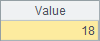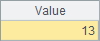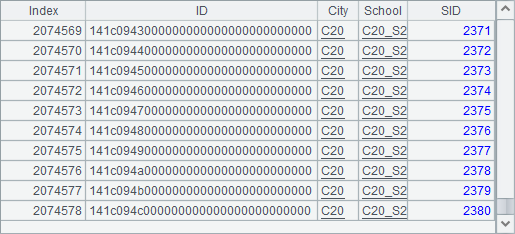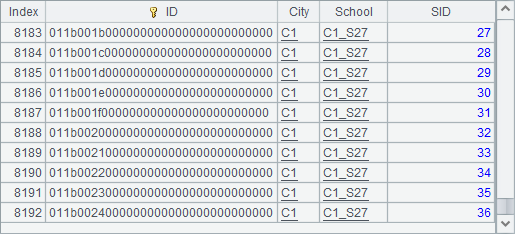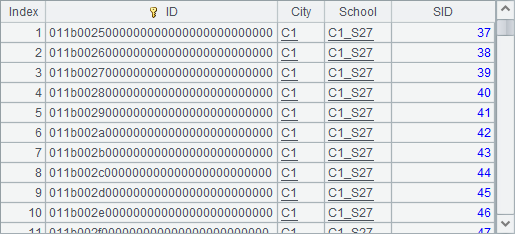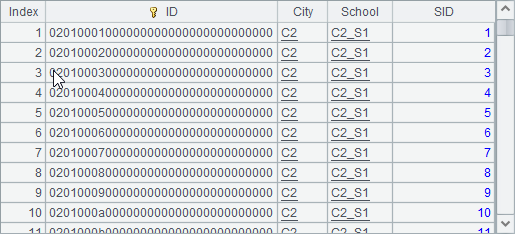## 柱图元的其它属性

Convex edge属性默认为false，如果设为true，则绘制柱图时采用突出边框效果。突出边框的效果通常用于纯色柱图，如下面的画法：

 A 1 =canvas() 2 =demo.query("select * from GYMSCORE") 3 =A1.plot("BackGround") 4 =A1.plot("EnumAxis","name":"x") 5 =A1.plot("NumericAxis","name":"y","location":2) 6 =A1.plot("Column","fillColor":[["ChartColor",0,false,-39271,-1,0],["ChartColor",0, false,-13210,-1,0],["ChartColor",0,false,-6697984,-1,0]],"axis1":"x","data1": A2.(EVENT+","+NAME),"axis2":"y","data2":A2.(SCORE)) 7 =A1.draw@p(450,200)

A6中柱图的填充属性部分如下：